TWO CONTINUOUS TRACES

Here the interaction of convergent and rectilinear systems is investigated to clarify how the convergent systems leave their imprint in the resultant lines even when they have been removed.
'Commencing with the simplest three line systems (x,y,z rectangular coordinates) laid out with pencil on a sheet, an erratic trace is followed using alternate x, y, z directions starting from an edge and returning to the margin at another point but carefully avoiding the point of convergence. A second trace playing against the first will emphasize this effect.'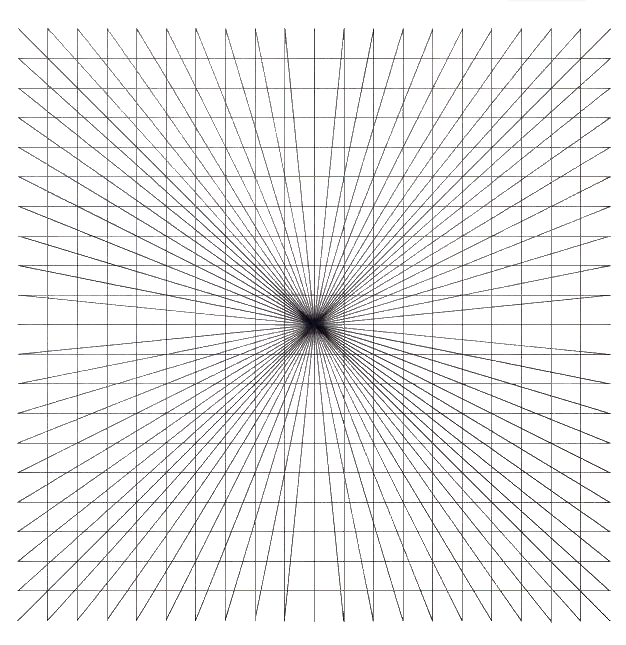.B. Rectangular coordinates system. Drawing executed with a computer
One has found in carrying this out with a computer that an extraordinary phenomenon arises by itself - namely that the space of the coordinate system curves around the center of the convergence - a mathematical reflexion of Einstein's idea that space is curved around matter.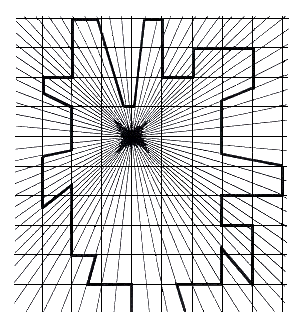Fig.62. One trace on a convergent axis and vertical and horizontal parallels
One continuous trace is made at random among the convergent system and the rectilinear system to give a heightened impression of centripetal forces acting on them both.This is stronger because they are interconnected within these systems.Fig.63. Two traces arising on a convergent axis and horizontal and vertical parallels.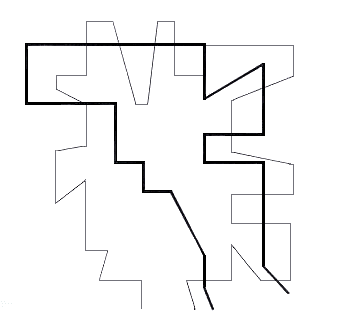Fig.64. Two traces extracted from figure 63
These two traces extracted from their coordinate systems provide an even stronger impression of being acted upon by centripetal force.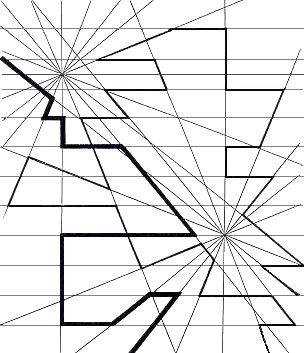Fig.65. Two continuous traces on two convergent axes and horizontal parallels
The two continuous traces are constructed at random following sections of the two converging systems and sections of parallel lines to give an impression of a strong irregular attraction.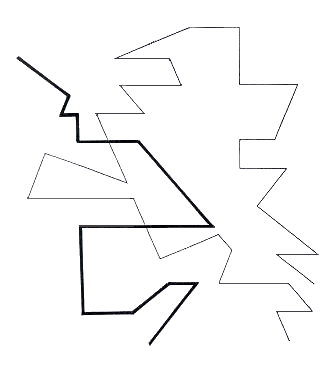Fig.66. The two traces extracted from figure 65
These two traces extracted from the two converging and parallel systems exhibit an attraction to the powerful centripetal forces mitigated by some parallel travelling.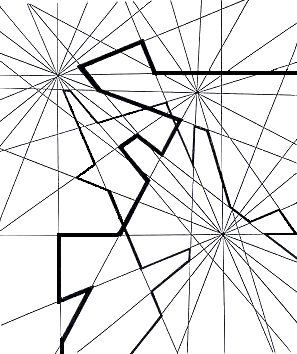Fig.67. Two traces on three convergent axes
Two continuous traces made at random among the converging systems give an almost violent impression of centripetal forces at odds with one another.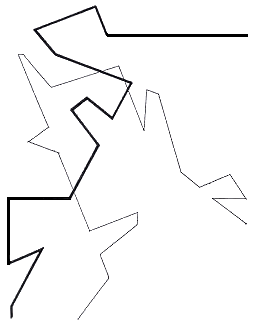Fig.68. The two traces extracted from figure 67
Two continuous traces extracted from their coordinate systems give a violent impression of competing attractive forces unmitigated by any parallel paths. These two continuous traces extracted from the framework of three converging systems suggest a number of centripetal centres acting (each partially determined by each) in a way that leads up to the situation of deterministic chaos.

In fact according to the laws of perspective, the single point of view is made for a single observer and he must be at a precise position to enjoy it. It is solipsistic. If there are more observers, each from his own point of view can enjoy another vanishing point and when he moves, the vanishing point moves with him. This is illustrated here with three vanishing points and one can see the interaction between them. However, it can be much more sophisticated as illustrated by the computer diagrams of fractals and systematic chaos, which had begun to interest Hayter toward the end of his life, in which an infinity of vanishing points, all articulated with reference to all of the others, is presented. This is a subtle interaction of all of the individual vanishing points with the whole.Image n°1. Computer representation of Deterministic Chaos 1
"Nr. 22/85",ESTHETIQUE DU CHAOS, Palais de la Découverte,1989 Paris, Germany
In this representation we can see a great number of vanishing points, but in an enlargement even more could actually be seen. One can go on and on enlarging and perceiving more vanishing points up to the point of the computer's resolution ability; this is an arbitrary given which could be improved. One can never in principle reach the final number which is a denumerable infinity, like the infinity of natural numbers.Image n°2. Computer representation of Deterministic Chaos 2
"Nr. 22/85",ESTHETIQUE DU CHAOS, Palais de la Découverte, 1989 Paris, Germany
This is an enlargement of Image n°1, wherein one can perceive many more vanishing points. This is an unfinished process rather than a definite counting.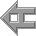back